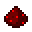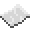# Bit32 API

Bit32.arshift Bit32.band Bit32.bnot Bit32.bor Bit32.btest Bit32.bxor Bit32.extract Bit32.lrotateFunctionbit32.lshift
Bitwise left-shift. When called, shifts all bits in a number the specified amount to the left. For example, 3 is 11 in binary. Shifting it two bits to the left makes it twelve (1100). A left-shift is also equivalent to number * 2shift.
Syntax bit32.lshift(
• number : number
• shift : number
)
Returns number shifted
Part of Lua (source)
API bit32ExampleShifting bits
Shifting ten (1010) 2 bits to the left. This should output 40, as 1010 becomes 101000
Code
```<nowiki>
local ten = 10
print(bit32.lshift(ten, 2))
</nowiki>
```
Output 40

Bit32.replace Bit32.rrotateFunctionbit32.rshift
Bitwise right-shift. When called, shifts all bits in a number the specified amount to the right. For example, twelve is 1100 in binary. Shifting it two bits to the right makes it 3 (11). A right-shift is also equivalent to number / 2shift.
Syntax bit32.rshift(
• number : number
• shift : number
)
Returns number shifted
Part of Lua (source)
API bit32ExampleShifting bits
Shifting ten (1010) 2 bits to the left. This should output 2, as 1010 becomes 10 (the last bit is dropped.)
Code
```<nowiki>
local ten = 10
print(bit32.rshift(ten, 2))
</nowiki>
```
Output 2